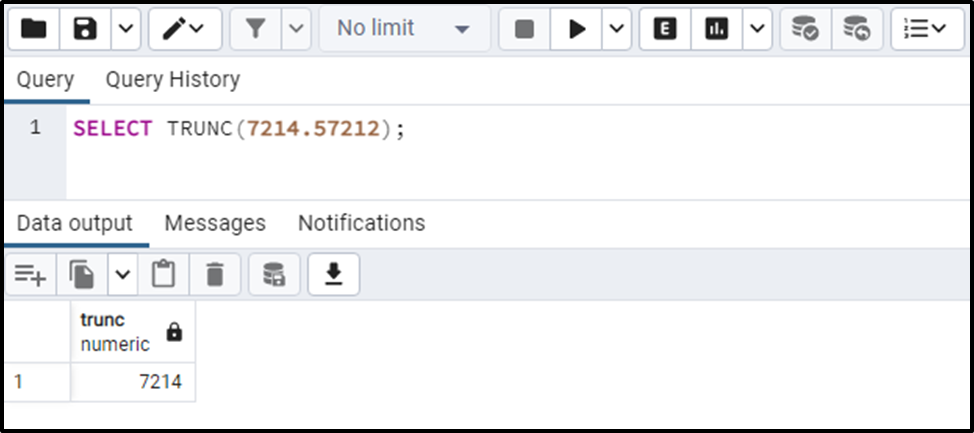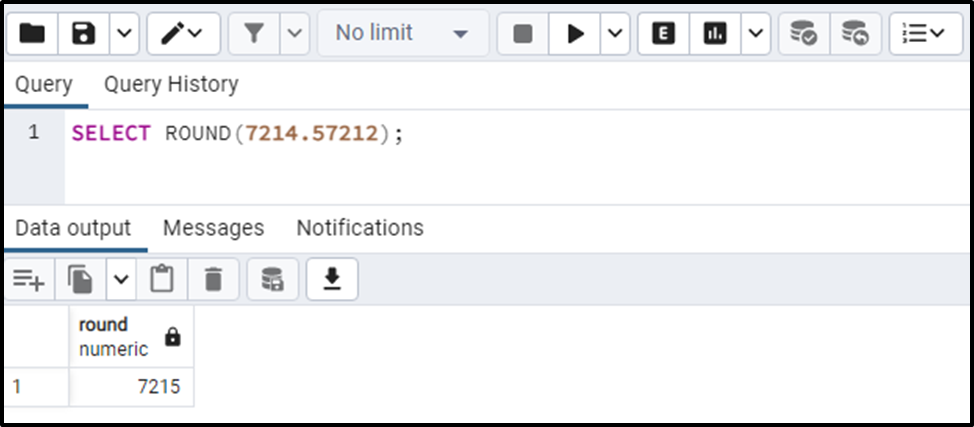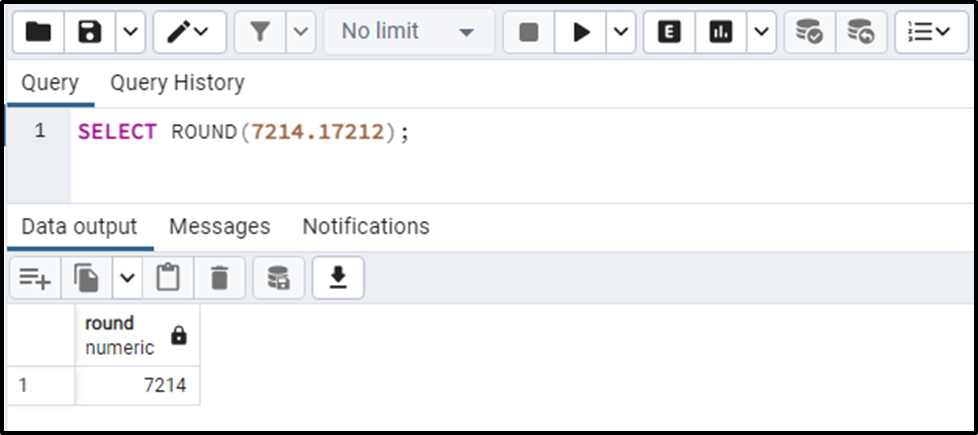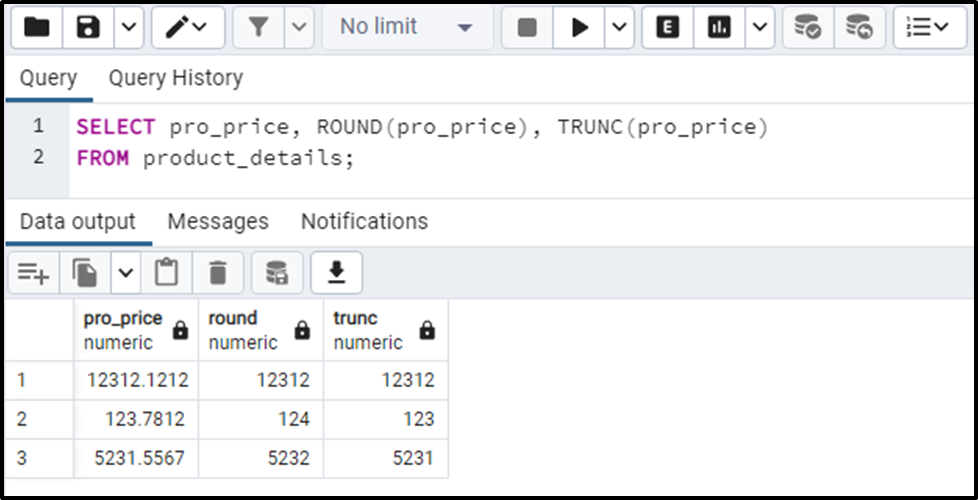##### PostgreSQL TRUNC() VS ROUND() Function

In PostgreSQL, the TRUNC() and ROUND() functions belong to the mathematical functions. The TRUNC() function trims the whole fractional part or up to specified precision, while the ROUND() function rounds the input number to the nearest integer/specified fractional places.

This blog post will compare the TRUNC(), and ROUND() functions through suitable examples. So, let’s begin.

PostgreSQL TRUNC() Function

In Postgres, the TRUNC() function accepts a numeric value as an argument, trims the fractional part, and retrieves the resultant integer:

`TRUNC(val_1, val_2);`

Here, the first argument indicates the input number, while the second argument determines the number of digits to be trimmed. Skipping the second argument will truncate the whole fractional part of the input number.

Example 1: TRUNC() Function With One Argument

If you pass only a single value, then the TRUNC() function will trim the whole fractional part:

`SELECT TRUNC(7214.57212);`From the output, you can observe that the fractional part has been trimmed completely.

Example 2: TRUNC() Function With Two Arguments

If you pass two values to the TRUNC() function, then the TRUNC() function will trim the input number based on the second value:

`SELECT TRUNC(7214.57212, 3);`

In the TRUNC() function, we specified ‘3’ as a second argument, so the input number will keep only three digits and skips the remaining fractional part:The output authenticates the working of the TRUNC() function.

PostgreSQL ROUND() Function

In Postgres, the ROUND() function accepts a numeric value as an argument, rounds the fractional part up to the nearest integer, and retrieves the resultant integer:

`ROUND(val_1, val_2);`

In the above syntax, the first argument represents the input number, while the second argument determines the number of digits to be rounded.

Note: The ROUND() function rounds the number nearest integer(upward if the fractional value is greater than or equal to the “.5” and downward if the value is less than “.5”)

Example 1: ROUND() Function With One Argument (>=.5)

Let’s pass a numeric value to the ROUND() function and see how it works:

`SELECT ROUND(7214.57212);`Since the fraction value was >= .5, therefore the ROUND() function rounds up the given number to the nearest integer.

Example 2: ROUND() Function With One Argument (<.5)

We will pass a numeric value “7214.17212” to the ROUND() function in this example:

`SELECT ROUND(7214.17212);`

The above snippet shows that the fractional part of the input value is less than “.5”. Let’s see how the ROUND() function work in such a situation:The above snippet shows that the ROUND() function rounded down the input number to the nearest integer.

PostgreSQL TRUNC() VS ROUND() Function

To understand the working of TRUNC() and ROUND() functions in a better way, let’s implement both these functions on the “pro_price” column of the product_details table:

```SELECT pro_price, ROUND(pro_price), TRUNC(pro_price)
FROM product_details;```The output snippet proves that the TRUNC() function trims the fractional part irrespective of what exactly it is. While the ROUND() function rounds the input number based on the fractional/decimal part(i.e., >= .5 or <.5).

Conclusion

TRUNC() and ROUND() are mathematical functions in PostgreSQL. The TRUNC() function trims the whole fractional part or up to specified precision, while the ROUND() function rounds the input number to the nearest integer/specified fractional places. The TRUNC() function trims the fractional part irrespective of what exactly it is(i.e., Greater than or less than .5). While the ROUND() function rounds the input number based on the fractional/decimal part. This write-up presented a comparative analysis of the Postgre TRUNC(), and ROUND() functions using practical examples.